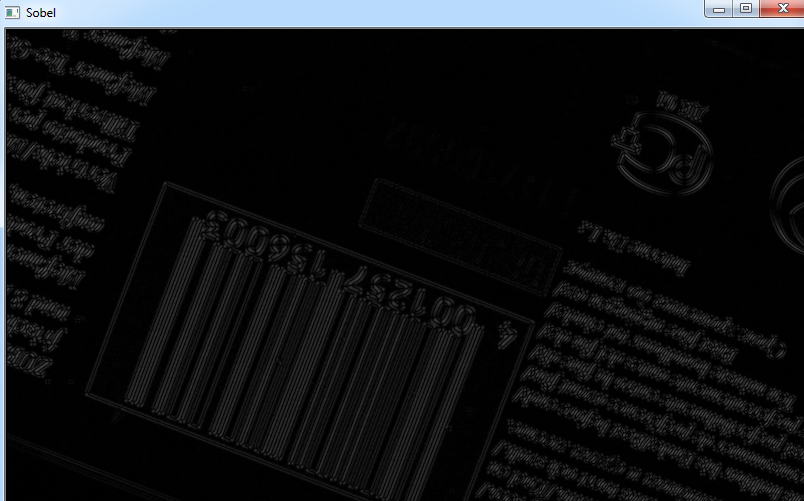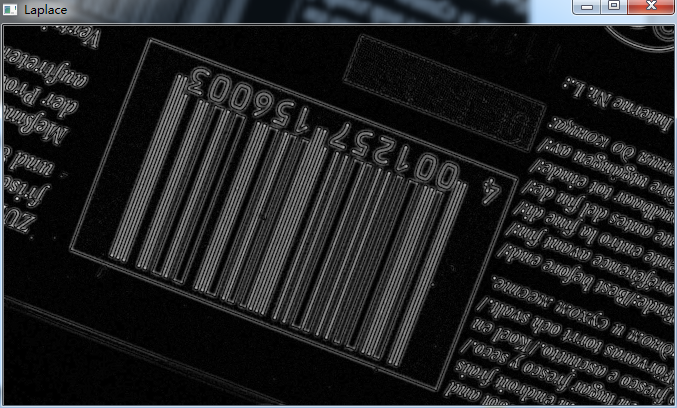×

# OpenCV Edge Detection

Jun 29, 2015

Changes or discontinuities of amplitude attribute, such as luminance value, are fundamentally important primitive characteristics of an image. They often provide an indication of the physical extent of objects. Local Discontinuities of image luminance that from one level to another are called luminance edges. In this post, I’ll share how to make image edge detection with OpenCV.

## Overview of Edge Detection

Edge detection is a very common task during image processing. OpenCV provides three most popular edge detection methods: Sobel, Canny, and Laplacian.

The Sobel function prototype is as follows:

``````CV_EXPORTS_W void Sobel( InputArray src, OutputArray dst, int ddepth,
int dx, int dy, int ksize = 3,
double scale = 1, double delta = 0,
int borderType = BORDER_DEFAULT );
``````

Canny is another famous edge detection algorithm. The function prototype is as follows:

``````CV_EXPORTS_W void Canny( InputArray image, OutputArray edges,
double threshold1, double threshold2,
int apertureSize = 3, bool L2gradient = false );
``````

Laplacian is a second-order derivative edge detection technique. The function prototype is as follows:

``````CV_EXPORTS_W void Laplacian( InputArray src, OutputArray dst, int ddepth,
int ksize = 1, double scale = 1, double delta = 0,
int borderType = BORDER_DEFAULT );
``````

## Building Image Edge Detection Application with Visual Studio 2013

1. Make sure to prepare your OpenCV environment successfully. If you want to build OpenCV lib by yourself, please refer to blog Building OpenCV with CMake on Windows.
2. Create a Win32 console project “EdgeDetectionDemo”.
3. Add Include directories of OpenCV:

`````` F:\opencv\build\include
F:\opencv\build\include\opencv2
``````
4. Add Library directories of OpenCV:

`````` F:\opencv\build\x86\vc12\lib
``````
5. Find Post-Build Event and add the following commands to copy relevant OpenCV DLLs:

`````` copy "F:\opencv\build\x86\vc12\bin\opencv_core2410.dll" \$(OutDir)
copy "F:\opencv\build\x86\vc12\bin\opencv_imgproc2410.dll" \$(OutDir)
copy "F:\opencv\build\x86\vc12\bin\opencv_highgui2410.dll" \$(OutDir)
``````
6. Use following code snippets:

`````` #include "imgproc/imgproc.hpp"
#include "highgui/highgui.hpp"

#ifdef _DEBUG // Change lib version: opencv_core{version}.lib
#pragma comment(lib, "opencv_core2410d.lib")
#pragma comment(lib, "opencv_imgproc2410d.lib")
#pragma comment(lib, "opencv_highgui2410d.lib")
#else
#pragma comment(lib, "opencv_core2410.lib")
#pragma comment(lib, "opencv_imgproc2410.lib")
#pragma comment(lib, "opencv_highgui2410.lib")
#endif

using namespace cv;

int main( int, char(( argv )
{
const char ( file_name = "f:\\test.jpg";

Mat src, src_gray;
int kernel_size = 3;
int scale = 1;
int delta = 0;
int ddepth = CV_16S;

const char( src_name = "Source";
const char( sobel_name = "Sobel";
const char( canny_name = "Canny";
const char( laplace_name = "Laplace";

cvtColor( src, src_gray, COLOR_RGB2GRAY );
GaussianBlur( src_gray, src_gray, Size(3,3), 0, 0, BORDER_DEFAULT );

namedWindow( src_name, WINDOW_AUTOSIZE );
namedWindow( sobel_name, WINDOW_AUTOSIZE );
namedWindow( canny_name, WINDOW_AUTOSIZE );
namedWindow( laplace_name, WINDOW_AUTOSIZE );

//This is for source image.
imshow(src_name, src);

//This is for sobel edge detection.
Mat sobel_dst;
Mat sobel_abs_dst;
Sobel(src_gray, sobel_dst, ddepth, 1, 1, kernel_size);
convertScaleAbs(sobel_dst, sobel_abs_dst);
imshow( sobel_name, sobel_abs_dst );

//This is for canny edge detection.
Mat canny_dst;
Canny(src_gray, canny_dst, 50, 200, 3, false);
imshow(canny_name, canny_dst);

//This is for laplace edge detection.
Mat laplace_dst;
Mat laplace_abs_dst;
Laplacian( src_gray, laplace_dst, ddepth, kernel_size, scale, delta, BORDER_DEFAULT );
convertScaleAbs( laplace_dst, laplace_abs_dst );
imshow( laplace_name, laplace_abs_dst);

waitKey(0);

return 0;
}
``````

## Edge Detection Showcase

a)  The source image.b) The edge image produced by sobel.c) The edge image produced by canny.d) The edge image produced by laplace.## Source Code

https://github.com/DynamsoftRD/opencv-programming/tree/master/edge-detecion